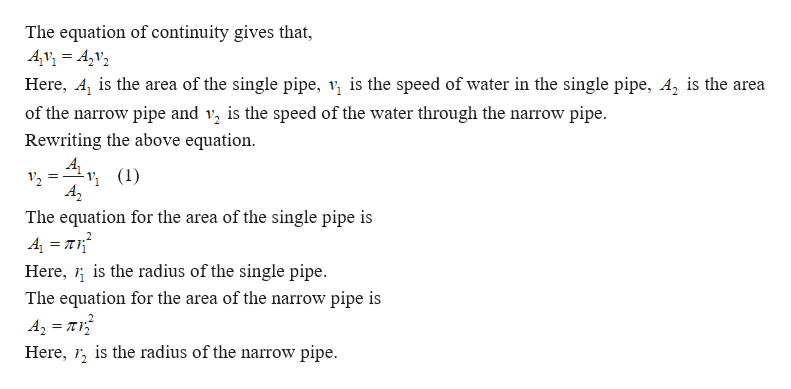# A man attaches a divider to an outdoor faucet so that water flows through a single pipe of radius 9.00 mm into two pipes, each with a radius of 6.00 mm. If water flows through the single pipe at 1.25 m/s, calculate the speed of the water in the narrower pipes.

Question
36 views

A man attaches a divider to an outdoor faucet so that water flows through a single pipe of radius 9.00 mm into two pipes, each with a radius of 6.00 mm. If water flows through the single pipe at 1.25 m/s, calculate the speed of the water in the narrower pipes.

check_circle

Step 1help_outlineImage TranscriptioncloseThe equation of continuity gives that, Av = A,v2 Here, A, is the area of the single pipe, v, is the speed of water in the single pipe, A, is the area of the narrow pipe and v, is the speed of the water through the narrow pipe. Rewriting the above equation. Av, (1) A, ½ (1) V2 The equation for the area of the single pipe is 4 = nr? Here, 1; is the radius of the single pipe. The equation for the area of the narrow pipe is A2 = T1 Here, 1, is the radius of the narrow pipe. fullscreen

### Want to see the full answer?

See Solution

#### Want to see this answer and more?

Solutions are written by subject experts who are available 24/7. Questions are typically answered within 1 hour.*

See Solution
*Response times may vary by subject and question.
Tagged in

### Fluid Mechanics